##### The Japanese Bonsai specialist
Direct order Contact Help / Services Newsletter# Copper watering can 2 litres

› Bonsai tools › Watering equipment › Copper watering can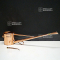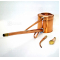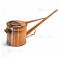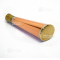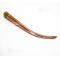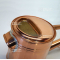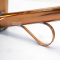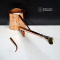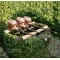ref. : 4338

135,00

This item is temporarily unavailableNotify availability

###### Description

Artisanal 2-litre copper watering can imported from Japan. Container: Ø 130 mm, height: 130 mm. Length of spout: 450 mm. Sold with one interchangeable rosette sprinkler head and one spout for precise watering. We recommend moderate watering for all bonsai.

Manufacturing process in Japan

#watering 7.8 #copper 5.6 #litres 2.6 #japan 2.5 #spout 2.5 #interchangeable 2.5 #manufacturing 2.3 #sprinkler 1.9 #recommend 1.9 #artisanal 1.9

Formule
(( ROUND((CHAR_LENGTH(b.article_nom)-CHAR_LENGTH(REPLACE(b.article_nom, 'watering', '')))/LENGTH('watering')) + ROUND((CHAR_LENGTH(b.article_description)-CHAR_LENGTH(REPLACE(b.article_description, 'watering', '')))/LENGTH('watering')) ) * 5.8) + (( ROUND((CHAR_LENGTH(b.article_nom)-CHAR_LENGTH(REPLACE(b.article_nom, 'copper', '')))/LENGTH('copper')) + ROUND((CHAR_LENGTH(b.article_description)-CHAR_LENGTH(REPLACE(b.article_description, 'copper', '')))/LENGTH('copper')) ) * 3.6) + (( ROUND((CHAR_LENGTH(b.article_nom)-CHAR_LENGTH(REPLACE(b.article_nom, 'litres', '')))/LENGTH('litres')) + ROUND((CHAR_LENGTH(b.article_description)-CHAR_LENGTH(REPLACE(b.article_description, 'litres', '')))/LENGTH('litres')) ) * 2.6) + (( ROUND((CHAR_LENGTH(b.article_nom)-CHAR_LENGTH(REPLACE(b.article_nom, 'japan', '')))/LENGTH('japan')) + ROUND((CHAR_LENGTH(b.article_description)-CHAR_LENGTH(REPLACE(b.article_description, 'japan', '')))/LENGTH('japan')) ) * 2.5) + (( ROUND((CHAR_LENGTH(b.article_nom)-CHAR_LENGTH(REPLACE(b.article_nom, 'spout', '')))/LENGTH('spout')) + ROUND((CHAR_LENGTH(b.article_description)-CHAR_LENGTH(REPLACE(b.article_description, 'spout', '')))/LENGTH('spout')) ) * 2.5) + (( ROUND((CHAR_LENGTH(b.article_nom)-CHAR_LENGTH(REPLACE(b.article_nom, 'interchangeable', '')))/LENGTH('interchangeable')) + ROUND((CHAR_LENGTH(b.article_description)-CHAR_LENGTH(REPLACE(b.article_description, 'interchangeable', '')))/LENGTH('interchangeable')) ) * 2.5) + (( ROUND((CHAR_LENGTH(b.article_nom)-CHAR_LENGTH(REPLACE(b.article_nom, 'manufacturing', '')))/LENGTH('manufacturing')) + ROUND((CHAR_LENGTH(b.article_description)-CHAR_LENGTH(REPLACE(b.article_description, 'manufacturing', '')))/LENGTH('manufacturing')) ) * 2.3) + (( ROUND((CHAR_LENGTH(b.article_nom)-CHAR_LENGTH(REPLACE(b.article_nom, 'sprinkler', '')))/LENGTH('sprinkler')) + ROUND((CHAR_LENGTH(b.article_description)-CHAR_LENGTH(REPLACE(b.article_description, 'sprinkler', '')))/LENGTH('sprinkler')) ) * 1.9) + (( ROUND((CHAR_LENGTH(b.article_nom)-CHAR_LENGTH(REPLACE(b.article_nom, 'recommend', '')))/LENGTH('recommend')) + ROUND((CHAR_LENGTH(b.article_description)-CHAR_LENGTH(REPLACE(b.article_description, 'recommend', '')))/LENGTH('recommend')) ) * 1.9) + (( ROUND((CHAR_LENGTH(b.article_nom)-CHAR_LENGTH(REPLACE(b.article_nom, 'artisanal', '')))/LENGTH('artisanal')) + ROUND((CHAR_LENGTH(b.article_description)-CHAR_LENGTH(REPLACE(b.article_description, 'artisanal', '')))/LENGTH('artisanal')) ) * 1.9)

## Secure payment## Delivery

Our logistic partners :04 74 55 23 48
Pépinière MAILLOT-BONSAÏ
Le Bois Frazy
01990 RELEVANT - FRANCE
on appointment